## ACT test prepthat actually works

### BRAIN-COMPATIBLE ACT PREP

Post Follow-up: Working the New ACT Math Probability Items

In the previous post, we divided the probability items found in the last two Preparing-for-the-ACT Guides into three categories. In this post, we work those items. If you have questions or comments, shoot me an email at kshipley@36university.com.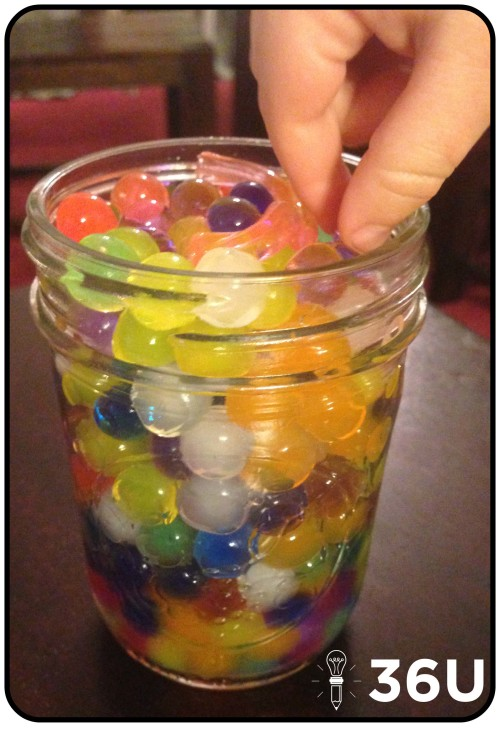These items are provided to give you examples of the ways you can expect ACT to test probability. These are screenshots from the ACT’s booklets, not the property of 36 University.

Item Type 1: Basic Probability Items

These items require you to apply the basic probability formula:

P(A) = (# of events corresponding to A/ total # of possible events)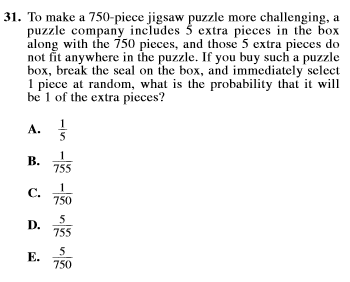#31 Solution:

Step 1) Recognize there are now 755 pieces.

Step 2) Of those 775 pieces, 5 are the new “extra” pieces.

Step 3) Use probability formula: P(extra piece) = 5/755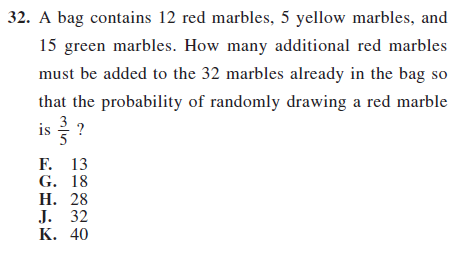#32 Solution:

Step 1) Current probability of red marble: 12/32

Step 2) Recognize we need a fraction that will reduce to 3/5, so the denominator has to reduce to 5.

Step 3) Begin adding red marbles in increments so that the total number of red marbles is divisible by 3.

Current Probability of red: 12/32 –> 3/8

Add 3 red marbles. Updated probability of drawing red marble: 15/35 –> 3/7

Add 8 red marbles. Updated probability of drawing red marble: 20/40 –> 1/2

Add 13 red marbles. Updated probability of drawing red marble: 25/45 –> 5/9

Add 18 red marbles. Updated probability of drawing red marble: 30/50 –> 3/5.  (I should have known!)

Item Type 2: Probability from Graphs

These items require you to utilize your graph reading skills and basic probability concepts.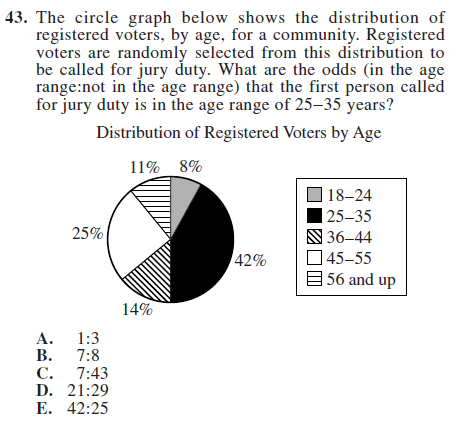#43 Solution:

Step 1) They provide formula for odds. Odds = in the age range:not in the age range.

Step 2) Percentage of persons in 25-35 years range: 42% or 42 out of 100.

Step 3) If 42 out of 100 are in the age range, the other 58 must not be in the age range.

Step 4) Set up the odds. 42:58 –> 21:29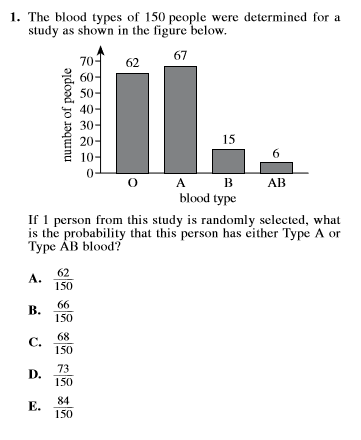#1 Solution:

Step 1: P(Type A or Type AB blood) = (# of Type A + # of Type AB)/ (Total # of possibilities)

Step 2: P(Type A or Type AB blood) = (67 + 6)/150 –> 73/150

Item Type 3: Probability of Multiple Events

Multiple events probability items ask you take one additional step. Often, that means you’ll just need to multiply the probabilities of each event. Here are examples: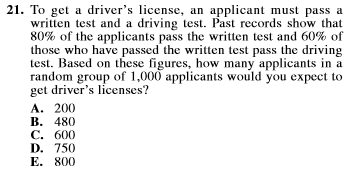#21 Solution:

Step 1: 80% pass written test. Of 1,000, we expect 800 to pass written test. (80% • 1000)

Step 2: Of those that pass written test, 60% pass driving test. 60% • 800 that passed written –> 480 expected to pass both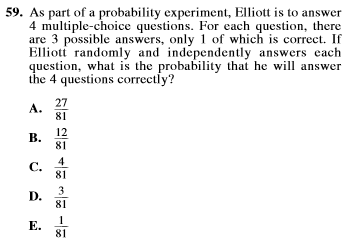#59 Solution:

Step 1: Chances of guessing a particular item correctly is 1/3, since there are three answer choices.

Step 2: Chances of guessing all 4 items correctly? Multiply the probabilities: (1/3) • (1/3) • (1/3) • (1/3) –> 1/81

Wrapping It Up

We hope these solutions helped you work these items more efficiently! If you have an alternative, quicker method to solving any of these items, we’d love to see it.

Recent & Related Posts:

3 Types of Probability Items to Expect on the ACT Math Test

Origami: Sharpen Visual-Spatial Skills & Boost Your ACT Math Score

Your Week-Before-the-ACT Game Plan (Part 4 of 4: Science)

36U ACT Tips

36U Blog List

36U ACT Prep Program

Three Types of Probability Items to Expect on the ACT Math Test

You walk into the testing site and sit down, nervous but confident. You know you’ve prepared and you’re ready to give the ACT one last shot. You are sure this will result in your best your score yet. The English test is no problem. You open the math test and begin working, but something has changed…Modifications to the ACT Math Test

Maybe you haven’t heard, but ACT announced test-takers could look forward to “the inclusion of additional statistics and probability items in the mathematics test” (Source: ACT FAQ). ACT hasn’t released volumes of materials on the changes, but the last two released exams contained in the Preparing-for-the-ACT Guides are consistent with the announced changes and provide the best clues we have on the amendments. We have divided the following items into three categories. The items are provided to give you examples of the ways you can expect ACT to test probability. These are screenshots from the ACT’s booklets, not the property of 36 University.

Item Type 1: Basic Probability Items

These items require you to apply the basic probability formula:

P(A) = (# of events corresponding to A/ total # of possible events)Item Type 2: Probability from Graphs

These items require you to utilize your graph reading skills and basic probability concepts.Item Type 3: Probability of Multiple Events

Multiple events probability items ask you take one additional step. Often, that means you’ll just need to multiply the probabilities of each event. Here are examples:Wrapping It Up

The good news is these items aren’t that complicated and are likely to replace more difficult items. These changes may mean it’s time for you to brush up on basic probability, especially since ACT may begin testing these concepts in more challenging ways.

Do you want to see these items worked? Check out our follow-up post. For more practice, check out the online Sample Items published by ACT. Specifically, try Set 3 Item #2.

Recent & Related Posts:

Post Follow-up: Working the New ACT Math Probability Items

Origami: Sharpen Visual-Spatial Skills & Boost Your ACT Math Score

Your Week-Before-the-ACT Game Plan (Part 4 of 4: Science)

Your Week-Before-the-ACT Game Plan (Part 3 of 4: Reading)

Your Week-Before-the-ACT Game Plan (Part 2 of 4: Math)

36U ACT Tips

36U Blog List

36U ACT Prep Program